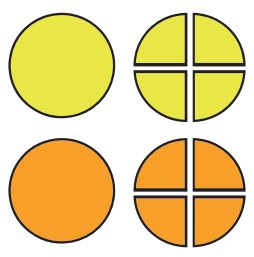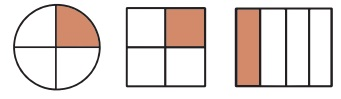Home | | Maths 4th Std | One fourth (quarter)

# One fourth (quarter)

Fractions : One fourth (quarter)

One fourth (quarter)Each fruit was divided into four equal quarters.Every pictures was divided into four equal parts. And shaded portion represent quarter or one fourth.

Tags : Fractions | Term 3 Chapter 6 | 4th Maths , 4th Maths : Term 3 Unit 6 : Fractions
Study Material, Lecturing Notes, Assignment, Reference, Wiki description explanation, brief detail
4th Maths : Term 3 Unit 6 : Fractions : One fourth (quarter) | Fractions | Term 3 Chapter 6 | 4th Maths